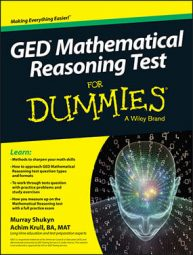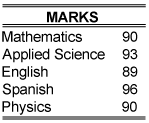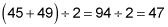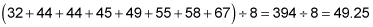##### GED Mathematical Reasoning Test For DummiesSome questions on the GED Mathematical Reasoning test may require you to give an answer based on a collection of data. You may need to find the mean, median, and mode, and use this information to answer the question.

In the following practice questions, you're asked to find the mean, median, and mode for a series of basketball scores, and to calculate a student's mean test score and compare it against the class median score.

## Practice questions

1. A junior league basketball team scored the following for its first 8 games: 45, 67, 55, 44, 32, 44, 58, and 49. Determine the mean, median, and mode for that series of numbers.

2. To be recommended for a scholarship at College of the Americas, a student must score above the median of all the students in his or her final year and have a mean mark of at least 90 percent. Delores is hoping for a scholarship recommendation with these marks:The median mark for the graduating students is 86.5. How many percentage points above the required minimum mean score did Delores score?

A. 1.6

B. 2.6

C. 3.0

D. 0.5

1. Mean: 49.25, Median: 47, Mode: 44

To answer the question, first put the values in order from least to greatest:

32, 44, 44, 45, 49, 55, 58, 67

You can instantly tell that the mode is 44 because it's the only number that appears more than once in the series. You have an even number of values, so to determine the median, you must add the two middle values and divide by two:And the mean is the average of all the values, so total the values and divide by 8:2. A.

1.6. Delores has a mean mark of 91.6, which is 1.6% above the minimum mean score.